Digits and Place Value: Part 1

 Problem Set 1 Problem Set 2 Problem Set 3Select a problem set using the buttons above, then use your mouse or tab key to select a question. Fill in the blank with the correct answer for each problem. When you have answered all of the questions, ask Charlie how you did.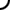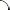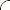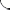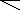For problems 1-6 use the number 8,204,719,365.   What digit is in the hundred thousands place? What digit is in the one billions place? What digit is in the hundreds place? What digit is in the ten millions place? What digit is in the ten thousands place? What digit is in the hundred millions place?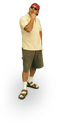For problems 1-6 use the number 1,395,204,867.   What digit is in the tens place? What digit is in the hundred millions place? What digit is in the thousands place? What digit is in the one billions place? What digit is in the ten thousands place? What digit is in the hundreds place?For problems 1-6 use the number 2,930,437,185.   What digit is in the one millions place? What digit is in the ones place? What digit is in the ten millions place? What digit is in the hundred thousands place? What digit is in the thousands place? What digit is in the tens place?©  2009  LarryPerezWeb Design:  Patrick Quigley